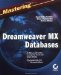# SQL Aggregate Functions

The earlier section, "The HAVING Clause," introduces that clause as a means of grouping data by a column or columns. Occasionally, you also want to summarize or otherwise evaluate the data in that column. You can do so using SQL Aggregate functions. Some systems have native functions that are similar and may or may not offer extended capabilities. You'll use the following SQL Aggregate functions to return statistical information on your grouped data. SQL Aggregate functions all accept one argument, which must be the reference to a column (field).

Avg(ALL | DISTINCT column) SQL's Avg() function returns the average or mean value of a group of values. For instance, the following statement returns the value 26.2185-the average unit price:SELECT Avg(UnitPrice) AS AvgPrice

FROM [Order Details]

The ALL predicate is the default; adding the DISTINCT keyword as follows returns the average value of 28.9207:

`SELECT Avg(DISTINCT UnitPrice) AS AvgPrice FROM [Order Details]`

Count(ALL | DISTINCT column | *) The Count() function returns the number of non-Null values in a group of values. The following statement returns the number of OrderID values in the Order Details table, which is 2155-the number of individual items, not orders:

`SELECT Count(OrderID) FROM [Order Details] `

Adding the DISTINCT predicate returns the number of orders, which is 830:

`SELECT Count(DISTINCT OrderID) FROM [Order Details]`

Using the asterisk character (*) instead of referencing an actual column returns the total number of rows in the underlying datasource. For instance, the following statement returns the number of employee records in the Employees table, which is 9:

`SELECT Count(*) FROM Employees`

In contrast, the following statement references a column that contains Null values and the Region value and returns the value 5:

`SELECT Count(Region) FROM Employees`

Sum(ALL | DISTINCT column) The Sum() function adds all the values in a group of values. For instance, the sum of all the UnitPrice entries in the Products table is \$2,222.71:

`SELECT Sum(UnitPrice) AS TotalPrice  FROM Products`

Adding the DISTINCT predicate changes the sum to \$1,948.81:

`SELECT Sum(DISTINCT UnitPrice) AS TotalPrice  FROM Products`

Min(column) The Min() function returns the smallest value in a group of values. For instance, the following statement returns the minimum UnitPrice entry of \$2.50:SELECT Min(UnitPrice) AS TotalPrice FROM Products

This function doesn't support the DISTINCT predicate because it considers only one value in its results.

Max(column) The Max() function is similar to the Min() function except it returns the largest value in a group of values. The following statement returns the value \$263.50:

`SELECT Max(UnitPrice) AS TotalPrice  FROM Products`

Similarly to the Min() function, the Max() function doesn't support the DISTINCT predicate.

StDev(column) The StDev() function estimates the standard deviation for a group of values.The following statement returns the standard deviation for freight, 44.41, in the RJ region:

`SELECT StDev(Freight) FROM Orders WHERE ShipRegion = 'RJ' `

StDevP(column) The StDevP() function evaluates the population for a group of values. For instance, the following statement returns the population for freight, 43.75, in the RJ region:

`SELECT StDevP(Freight) FROM Orders WHERE ShipRegion = 'RJ'`

Var(column) The Var() function estimates variance. For instance, the following statement estimates the variance for freight, 1971.85, on orders shipped to the RJ region:

`SELECT Var(Freight) FROM Orders WHERE ShipRegion = 'RJ'`

VarP(column) The VarP() function evaluates the variance by population. The following statement evaluates the variance across the population for the freight, 1913.86, shipped to the RJ region:

`SELECT VarP(Freight)`

FROM Orders

WHERE ShipRegion = 'RJ'Nowadays developers wear many hats, and the luxury of specializing in one language is long gone. SQL is probably the one skill that all web developers share because you must use SQL to interact with the database. You must do more than learn the basics; you must become proficient at SQL. This chapter has served as an introduction only. We encourage you to explore further sources of information on your own. One of the best sources of information about all aspects of SQL is Martin Gruber's Mastering SQL (Sybex, 2000). Another good choice for anyone working with Microsoft Access and SQL Server is SQL: Access to SQL Server, by Susan Sales Harkins and Martin W. P. Reid (Apress, 2002).Mastering Dreamweaver MX Databases
ISBN: 078214148X
EAN: 2147483647
Year: 2002
Pages: 214